# Octave Basics: Solving Systems of Linear Equations

## Goal: Solve a system of Linear Equations

The OS used in this tutorial is Manjaro linux, This tutorial uses terminal and should work on ALL operating systems.

To open up Octave, open up your terminal and type 'octave'. This prompts you with a screen of credits, followed by 'octave:1>'

Let's compose a three dimensional matrix equation, which we will call 'A'

A would be written as so like so: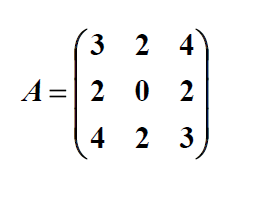In terminal, we can write this as A = [3, 2, 4; 2, 0, 2; 4, 2, 3]

If we type 'A' in terminal, the matrix we inputted should be displayed see image be below: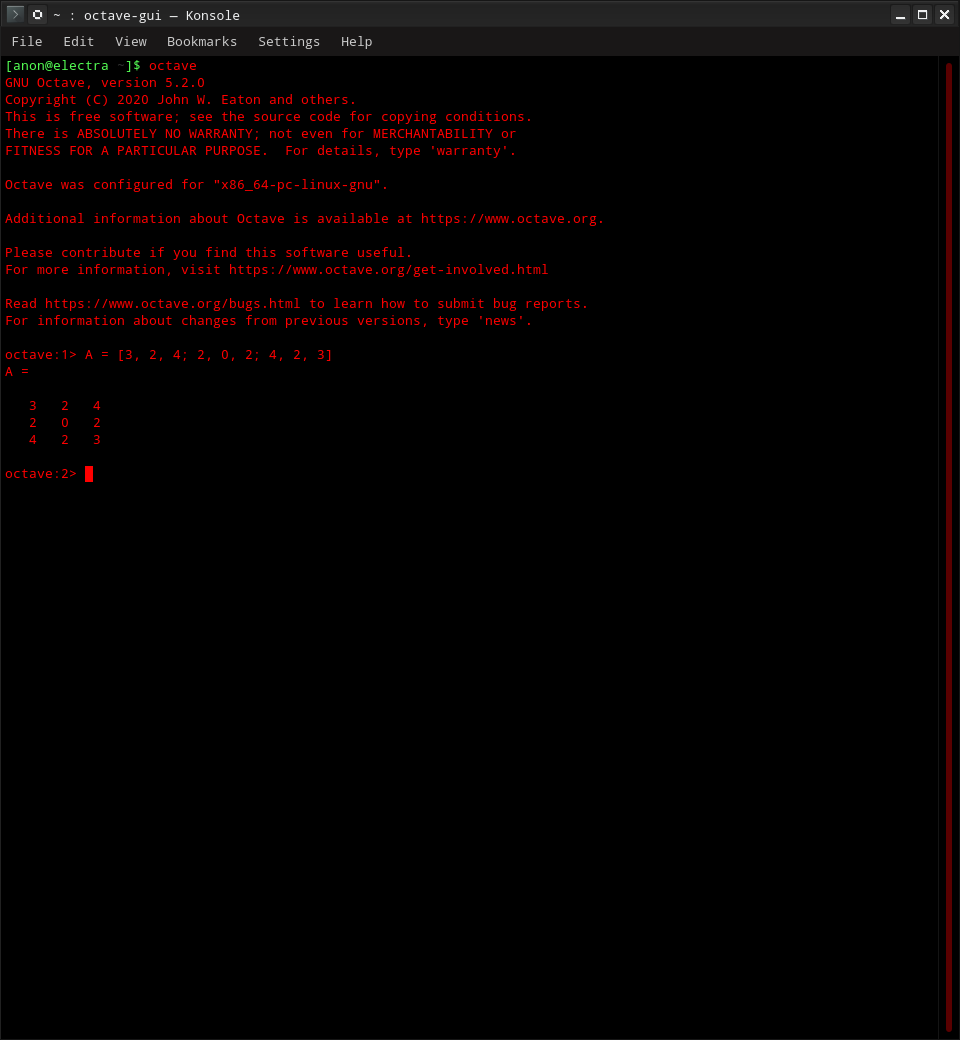Now we have matrix 'A' in the memory of our terminal session

We will now establish matrix 'b', which is a 3x1 matrix.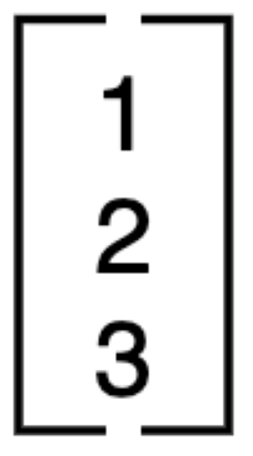Establish matrix 'b' using the same syntax we did with 'A', and recall the matrxiWe will now compute unknown variables x1, x2, and x3. A written representation is in the picture below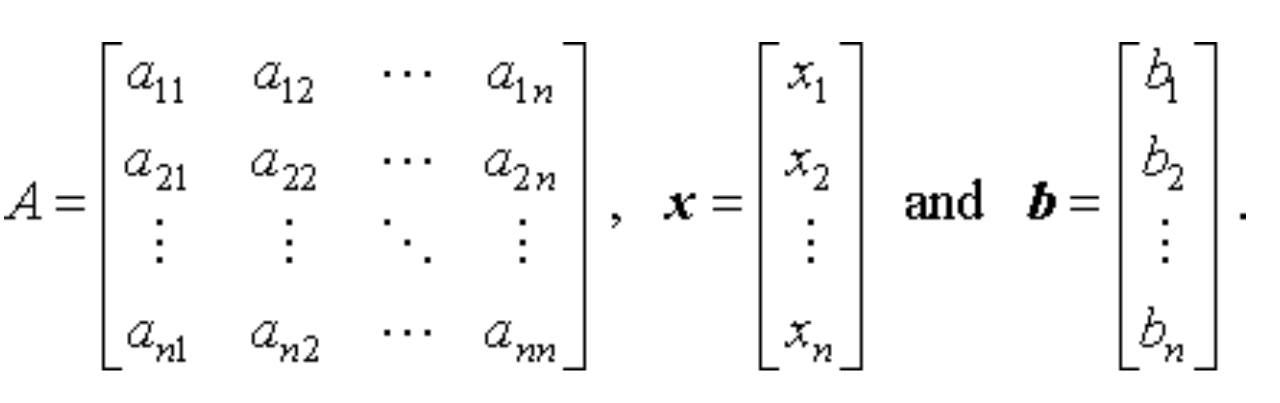We can compute the unknown variables by recalling the two matrices we've composed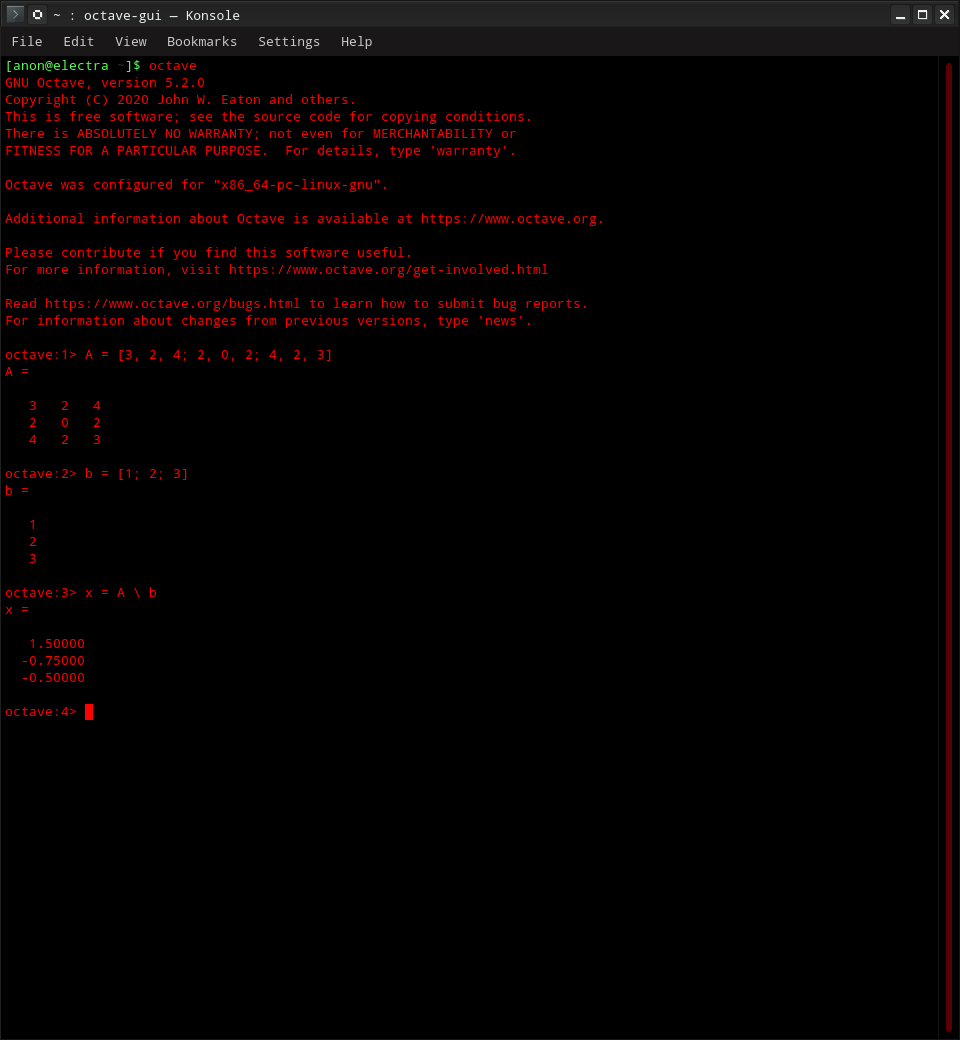### Conclusion

We have solved all Unknown variables. We have:

• x1 = 1.50
• x2 = -0.75
• x3 = -0.50